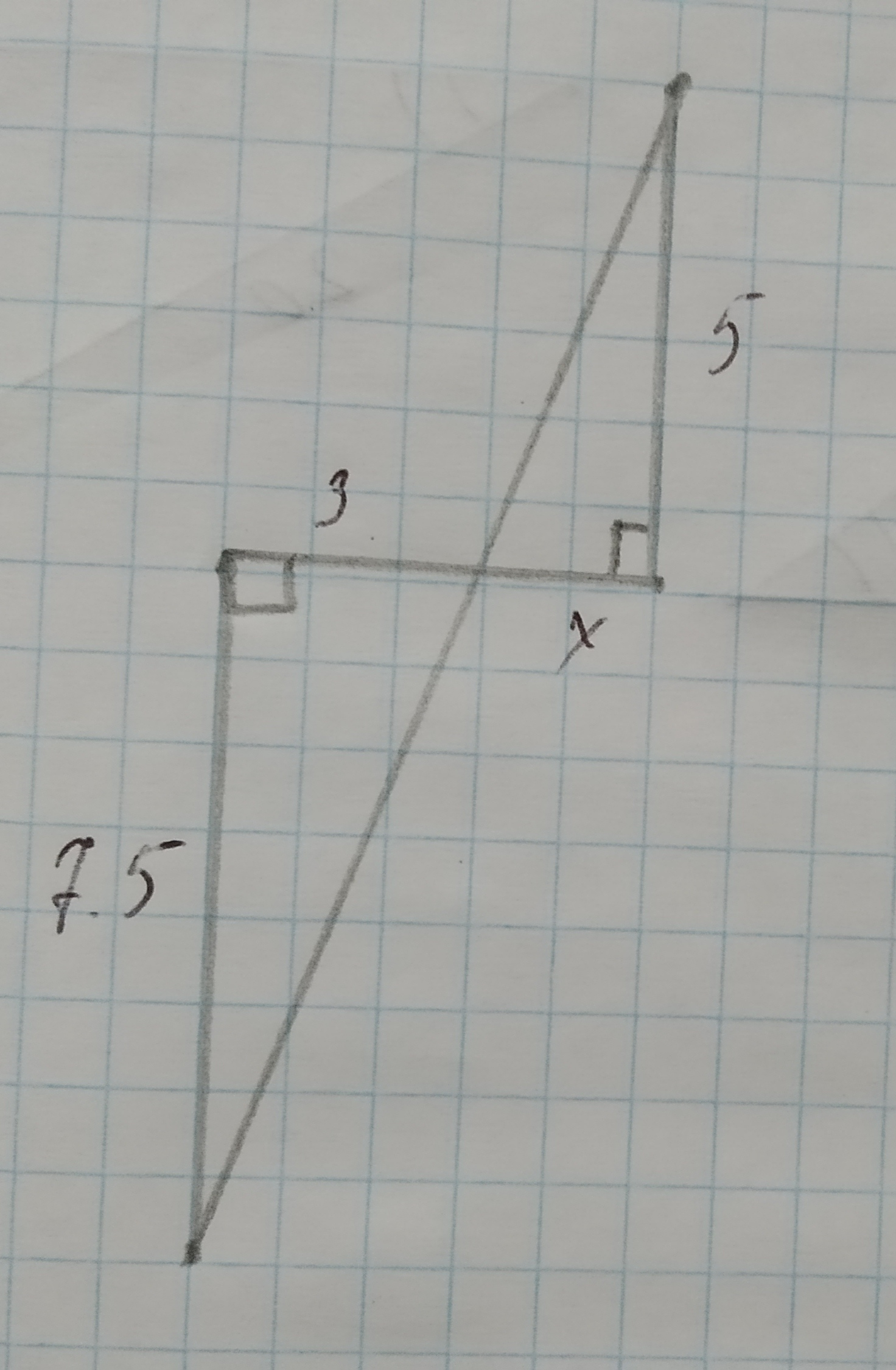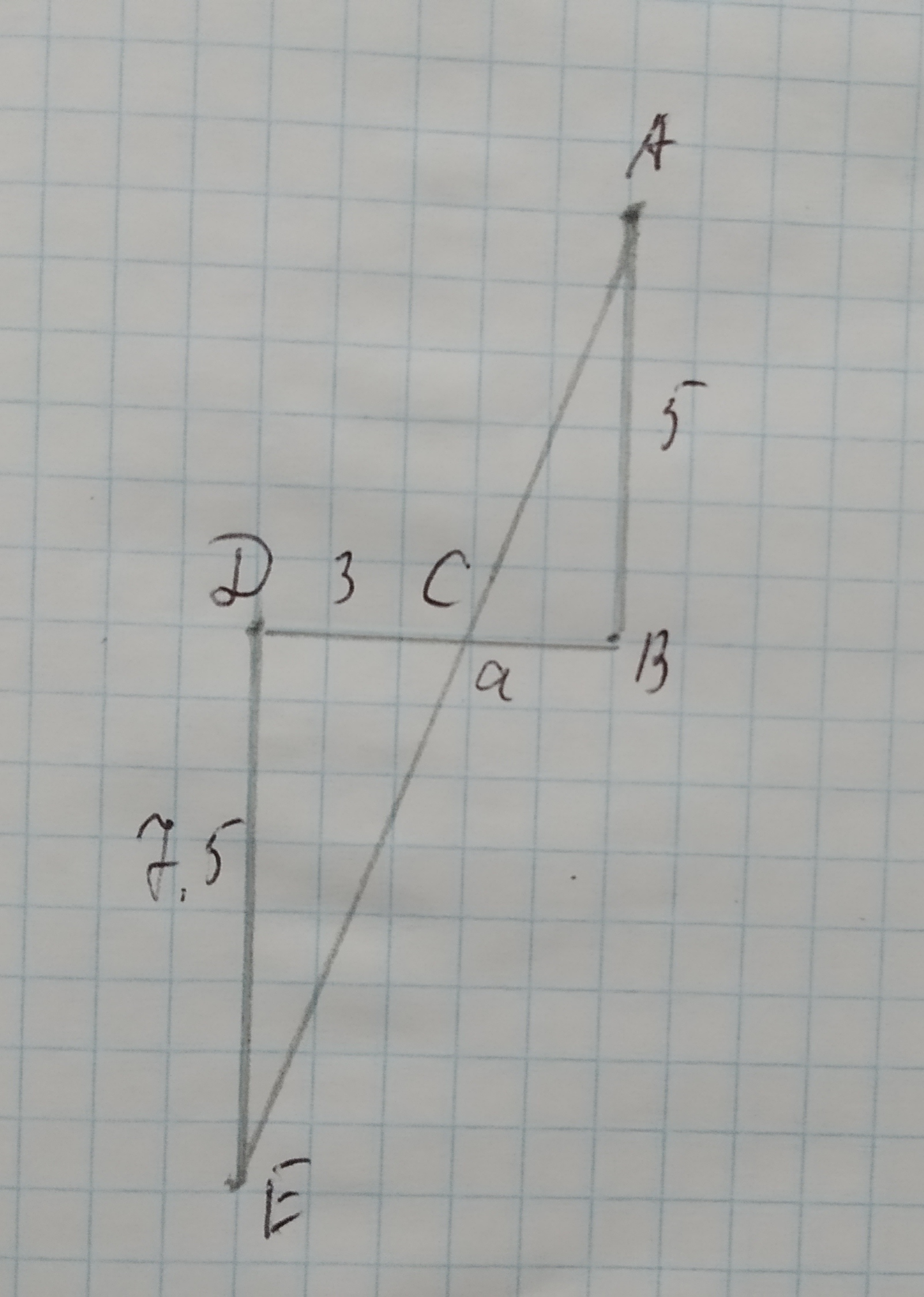# Find the length x.12210107131.jpgnagasenaz 2020-10-18 Answered

Find the length x.You can still ask an expert for help

• Questions are typically answered in as fast as 30 minutes

Solve your problem for the price of one coffee

• Math expert for every subject
• Pay only if we can solve itAlannej

Step 1
Solving by similarity of triangle
Step 2$9n\mathrm{△}ABC\phantom{\rule{1em}{0ex}}\text{and}\phantom{\rule{1em}{0ex}}\mathrm{△}CDE$,
$m (Given)
$\phantom{\rule{1em}{0ex}}\text{and}\phantom{\rule{1em}{0ex}}m
So $\mathrm{△}ABC$ is similar to $\mathrm{△}CDE$.
$⇒\frac{AB}{DE}=\frac{CB}{CD}$
$⇒\frac{5}{7\cdot 5}=\frac{x}{3}$
$⇒x=\frac{15}{7\cdot 5}=2$
x=2